Home
Hostname: page-component-55597f9d44-mm7gn Total loading time: 1.065 Render date: 2022-08-09T21:05:55.243Z Has data issue: true Feature Flags: { "shouldUseShareProductTool": true, "shouldUseHypothesis": true, "isUnsiloEnabled": true, "useRatesEcommerce": false, "useNewApi": true } hasContentIssue true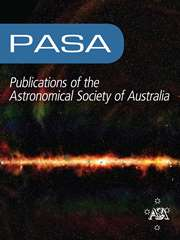Publications of the Astronomical Society of Australia

## Abstract

We illustrate the extraordinary discovery potential for extragalactic astrophysics of a far-infrared/submillimetre (far-IR/submm) all-sky spectroscopic survey with a 3-m-class space telescope. Spectroscopy provides a three-dimensional view of the Universe and allows us to take full advantage of the sensitivity of present-day instrumentation, close to fundamental limits, overcoming the spatial confusion that affects broadband far-IR/submm surveys. A space telescope of the 3-m class (which has already been described in recent papers) will detect emission lines powered by star formation in galaxies out to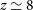$z\,{\simeq}\,8$ . It will specifically provide measurements of spectroscopic redshifts, star-formation rates (SFRs), dust masses, and metal content for millions of galaxies at the peak epoch of cosmic star formation and of hundreds of them at the epoch of reionisation. Many of these star-forming galaxies will be strongly lensed; the brightness amplification and stretching of their sizes will make it possible to investigate (by means of follow-up observations with high-resolution instruments like ALMA, JWST, and SKA) their internal structure and dynamics on the scales of giant molecular clouds (40–100 pc). This will provide direct information on the physics driving the evolution of star-forming galaxies. Furthermore, the arcmin resolution of the telescope at submm wavelengths is ideal for detecting the cores of galaxy proto-clusters, out to the epoch of reionisation. Due to the integrated emission of member galaxies, such objects (as well as strongly lensed sources) will dominate at the highest apparent far-IR luminosities. Tens of millions of these galaxy-clusters-in-formation will be detected at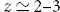$z \simeq 2 - 3$ –3, with a tail extending out to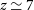$z\,{\simeq}\,7$ , and thousands of detections at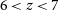$6\,{<}\,z\,{<}\,7$ . Their study will allow us to track the growth of the most massive halos well beyond what is possible with classical cluster surveys (mostly limited to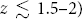$z\,\lesssim\, 1.5 - 2$ –2), tracing the history of star formation in dense environments and teaching us how star formation and galaxy-cluster formation are related across all epochs. The obscured cosmic SFR density of the Universe will thereby be constrained. Such a survey will overcome the current lack of spectroscopic redshifts of dusty star-forming galaxies and galaxy proto-clusters, representing a quantum leap in far-IR/submm extragalactic astrophysics.

Type
Research Article

## Access options

Get access to the full version of this content by using one of the access options below. (Log in options will check for institutional or personal access. Content may require purchase if you do not have access.)

## References

Abazajian, K., et al. 2019, arXiv e-prints, p. arXiv:1907.04473Google Scholar
Alberts, S., et al. 2014, MNRAS, 437, 437CrossRefGoogle Scholar
Alberts, S., et al. 2016, ApJ, 825, 72CrossRefGoogle Scholar
Bakx, T. J. L. C., et al. 2018, MNRAS, 473, 1751CrossRefGoogle Scholar
Barger, A. J., Cowie, L. L., Sanders, D. B., Fulton, E., Taniguchi, Y., Sato, Y., Kawara, K., & Okuda, H. 1998, Nature, 394, 248CrossRefGoogle Scholar
Barger, A. J., Cowie, L. L., Smail, I., Ivison, R. J., Blain, A. W., & Kneib, J. P. 1999, AJ, 117, 2656CrossRefGoogle Scholar
Basu, K., et al. 2019, arXiv e-prints, p. arXiv:1909.01592Google Scholar
Baugh, C. M., Lacey, C. G., Frenk, C. S., Granato, G. L., Silva, L., Bressan, A., Benson, A. J., & Cole, S. 2005, MNRAS, 356, 1191CrossRefGoogle Scholar
Benitez, N., et al. 2014, arXiv e-prints, p. arXiv:1403.5237Google Scholar
Bernardi, M., Shankar, F., Hyde, J. B., Mei, S., Marulli, F., & Sheth, R. K. 2010, MNRAS, 404, 2087Google Scholar
Bethermin, M., et al. 2020, arXiv e-prints, p. arXiv:2002.00962Google Scholar
Biggs, A. D., Younger, J. D., & Ivison, R. J. 2010, MNRAS, 408, 342CrossRefGoogle Scholar
Blain, A. W., & Longair, M. S. 1993, MNRAS, 264, 509CrossRefGoogle Scholar
Bleem, L. E., et al. 2020, ApJS, 247, 25CrossRefGoogle Scholar
Bonato, M., et al. 2019, PASA, 36, e017CrossRefGoogle Scholar
Boselli, A., et al. 2016, A&A, 596, A11Google Scholar
Bothwell, M. S., et al. 2013, MNRAS, 429, 3047CrossRefGoogle Scholar
Boylan-Kolchin, M., Springel, V., White, S. D. M., Jenkins, A., & Lemson, G. 2009, MNRAS, 398, 1150CrossRefGoogle Scholar
Cañameras, R., et al. 2015, A&A, 581, A105Google Scholar
Cañameras, R., et al. 2017, A&A, 604, A117Google Scholar
Cañameras, R., et al. 2018, A&A, 620, A60Google Scholar
Cai, Z.-Y., et al. 2013, ApJ, 768, 21CrossRefGoogle Scholar
Cai, Z.-Y., Lapi, A., Bressan, A., De Zotti, G., Negrello, M., & Danese, L. 2014, ApJ, 785, 65CrossRefGoogle Scholar
Cai, Z.-Y., De Zotti, G., & Bonato, M. 2020, ApJ, 891, 74CrossRefGoogle Scholar
Carniani, S., et al. 2017, A&A, 605, A42Google Scholar
Carniani, S., et al. 2018, MNRAS, 478, 1170CrossRefGoogle Scholar
Casey, C. M., et al. 2012a, ApJ, 761, 139CrossRefGoogle Scholar
Casey, C. M., et al. 2012b, ApJ, 761, 140CrossRefGoogle Scholar
Casey, C. M., Narayanan, D., & Cooray, A. 2014, Phys. Rep., 541, 45CrossRefGoogle Scholar
Casey, C. M., et al. 2015, ApJL, 808, L33CrossRefGoogle Scholar
Chapman, S. C., Blain, A. W., Smail, I., & Ivison, R. J. 2005, ApJ, 622, 772CrossRefGoogle Scholar
Chen, C.-C., et al. 2016, ApJ, 831, 91CrossRefGoogle Scholar
Chluba, J., et al. 2019, arXiv e-prints, p.arXiv:1909.01593Google Scholar
Clements, D. L., et al. 2016, MNRAS, 461, 1719CrossRefGoogle Scholar
Cooke, E. A., et al. 2018, ApJ, 861, 100CrossRefGoogle Scholar
Cutri, R. M., et al. 2013, Technical report, Explanatory Supplement to the AllWISE Data Release ProductsGoogle Scholar
Danielson, A. L. R., et al. 2017, ApJ, 840, 78CrossRefGoogle Scholar
Dannerbauer, H., Lehnert, M. D., Lutz, D., Tacconi, L., Bertoldi, F., Carilli, C., Genzel, R., & Menten, K. 2002, ApJ, 573, 473CrossRefGoogle Scholar
Dannerbauer, H., Lehnert, M. D., Lutz, D., Tacconi, L., Bertoldi, F., Carilli, C., Genzel, R., & Menten, K. M. 2004, ApJ, 606, 664CrossRefGoogle Scholar
Dannerbauer, H., Walter, F., & Morrison, G. 2008, ApJL, 673, L127CrossRefGoogle Scholar
Dannerbauer, H., et al. 2014, A&A, 570, A55Google Scholar
Dannerbauer, H., et al. 2019a, BAAS, 51, 293Google Scholar
Dannerbauer, H., Harrington, K., Daz-Sánchez, A., Iglesias-Groth, S., Rebolo, R., Genova-Santos, R. T., & Krips, M. 2019b, AJ, 158, 34CrossRefGoogle Scholar
De Looze, I., et al. 2014, A&A, 568, A62Google Scholar
Delabrouille, J., et al. 2019, arXiv e-prints, p.arXiv:1909.01591Google Scholar
Díaz-Sanchez, A., Iglesias-Groth, S., Rebolo, R., & Dannerbauer, H. 2017, ApJL, 843, L22CrossRefGoogle Scholar
Dressler, A. 1980, ApJ, 236, 351CrossRefGoogle Scholar
Dudzevičiūtė, U., et al. 2020, MNRAS, 494, 3828CrossRefGoogle Scholar
Dunlop, J. S., et al. 2004, MNRAS, 350, 769CrossRefGoogle Scholar
Eales, S., et al. 2010, PASP, 122, 499CrossRefGoogle Scholar
Flores-Cacho, I., et al. 2016, A&A, 585, A54Google Scholar
Franceschini, A., Toffolatti, L., Mazzei, P., Danese, L., & de Zotti, G. 1991, A&A Supp., 89, 285Google Scholar
Fu, H., et al. 2012, ApJ, 753, 134CrossRefGoogle Scholar
Fudamoto, Y., et al. 2017, MNRAS, 472, 2028CrossRefGoogle Scholar
Fujimoto, S., et al. 2019, ApJ, 887, 107CrossRefGoogle Scholar
Gobat, R., et al. 2011, A&A, 526, A133Google Scholar
Gómez-Guijarro, C., et al. 2019, ApJ, 872, 117CrossRefGoogle Scholar
Gonzalez, A. H., et al. 2019, ApJS, 240, 33CrossRefGoogle Scholar
Grandis, S., Mohr, J. J., Dietrich, J. P., Bocquet, S., Saro, A., Klein, M., Paulus, M., & Capasso, R. 2019, MNRAS, 488, 2041Google Scholar
Gruppioni, C., et al. 2013, MNRAS, 432, 23CrossRefGoogle Scholar
Gruppioni, C., et al. 2015, MNRAS, 451, 3419CrossRefGoogle Scholar
Gullberg, B., et al. 2015, MNRAS, 449, 2883CrossRefGoogle Scholar
Hanany, S., et al. 2019, arXiv e-prints, p.arXiv:1902.10541Google Scholar
Harrington, K. C., et al. 2016, MNRAS, 458, 4383CrossRefGoogle Scholar
Harrington, K. C., et al. 2018, MNRAS, 474, 3866CrossRefGoogle Scholar
Hashimoto, T., et al. 2018, Nature, 557, 392CrossRefGoogle Scholar
Heckman, T. M., & Best, P. N. 2014, ARA&A, 52, 589CrossRefGoogle Scholar
Hezaveh, Y. D., et al. 2013, ApJ, 767, 132CrossRefGoogle Scholar
Higuchi, R., et al. 2019, ApJ, 879, 28CrossRefGoogle Scholar
Hill, R., et al. 2020, arXiv e-prints, p.arXiv:2002.11600Google Scholar
Hilton, M.et al., 2018, ApJS, 235, 20CrossRefGoogle Scholar
Hughes, D. H., et al. 1998, Nature, 394, 241CrossRefGoogle Scholar
Hung, C.-L., et al. 2016, ApJ, 826, 130CrossRefGoogle Scholar
Inoue, A. K., et al. 2016, Science, 352, 1559CrossRefGoogle Scholar
Ivison, R. J., Smail, I., Le Borgne, J. F., Blain, A. W., Kneib, J. P., Bezecourt, J., Kerr, T. H., & Davies, J. K. 1998, MNRAS, 298, 583CrossRefGoogle Scholar
Ivison, R. J., et al. 2002, MNRAS, 337, 1CrossRefGoogle Scholar
Ivison, R. J., et al. 2013, ApJ, 772, 137CrossRefGoogle Scholar
Ivison, R. J., Page, M. J., Cirasuolo, M., Harrison, C. M., Mainieri, V., Arumugam, V., & Dudzevičiūtė, U. 2019, MNRAS, p. 2100Google Scholar
Jones, G. C., Maiolino, R., Caselli, P., & Carniani, S. 2019, A&A, 632, L7Google Scholar
Kato, Y., et al. 2016, MNRAS, 460, 3861CrossRefGoogle Scholar
Kauffmann, G., White, S. D. M., Heckman, T. M., Ménard, B., Brinchmann, J., Charlot, S., Tremonti, C., & Brinkmann, J. 2004, MNRAS, 353, 713CrossRefGoogle Scholar
Kaviani, A., Haehnelt, M. G., & Kauffmann, G. 2003, MNRAS, 340, 739CrossRefGoogle Scholar
Kennicutt, R. C., & Evans, N. J. 2012, ARA&A, 50, 531CrossRefGoogle Scholar
King, A., & Pounds, K. 2015, ARA&A, 53, 115CrossRefGoogle Scholar
Klein, M., et al. 2019, MNRAS, 488, 739CrossRefGoogle Scholar
Kneissl, R., et al. 2019, A&A, 625, A96Google Scholar
Kravtsov, A. V., & Borgani, S. 2012, ARA&A, 50, 353CrossRefGoogle Scholar
Kubo, M., et al. 2019, ApJ, 887, 214CrossRefGoogle Scholar
LSST Science Collaboration 2009, arXiv e-prints, p.arXiv:0912.0201Google Scholar
Lacaille, K. M., et al. 2019, MNRAS, 488, 1790CrossRefGoogle Scholar
Laporte, N., et al. 2017, ApJL, 837, L21CrossRefGoogle Scholar
Laureijs, R., et al. 2011, arXiv e-prints, p.arXiv:11http://dx.doi.org/10.3193Google Scholar
Lemaux, B. C., et al. 2019, MNRAS, 490, 1231CrossRefGoogle Scholar
Lima, M., Jain, B., & Devlin, M. 2010, MNRAS, 406, 2352CrossRefGoogle Scholar
Lupu, R. E., et al. 2012, ApJ, 757, 135CrossRefGoogle Scholar
Lutz, D. 2014, ARA&A, 52, 373CrossRefGoogle Scholar
Magnelli, B., et al. 2013, A&A, 553, A132Google Scholar
Mancuso, C., et al. 2015, ApJ, 810, 72CrossRefGoogle Scholar
Massardi, M., et al. 2018, A&A, 610, A53Google Scholar
Meixner, M., et al. 2019, arXiv e-prints, p.arXiv:1912.06213Google Scholar
Miller, T. B., et al. 2018, Nature, 556, 469CrossRefGoogle Scholar
Mocanu, L. M., et al. 2013, ApJ, 779, 61CrossRefGoogle Scholar
Nantais, J. B., et al. 2017, MNRAS, 465, L104CrossRefGoogle Scholar
Nayyeri, H., et al. 2016, ApJ, 823, 17CrossRefGoogle Scholar
Negrello, M., González-Nuevo, J., Magliocchetti, M., Moscardini, L., De Zotti, G., Toffolatti, L., & Danese, L. 2005, MNRAS, 358, 869CrossRefGoogle Scholar
Negrello, M., Perrotta, F., González-Nuevo, J., Silva, L., de Zotti, G., Granato, G. L., Baccigalupi, C., & Danese, L. 2007, MNRAS, 377, 1557CrossRefGoogle Scholar
Negrello, M., et al. 2010, Science, 330, 800CrossRefGoogle Scholar
Negrello, M., et al. 2014, MNRAS, 440, 1999CrossRefGoogle Scholar
Negrello, M.et al. 2017a, MNRAS, 465, 3558CrossRefGoogle Scholar
Negrello, M., et al. 2017b, MNRAS, 470, 2253CrossRefGoogle Scholar
Neri, R., et al. 2020, A&A, 635, A7Google Scholar
Nesvadba, N. P. H., Cañameras, R., Kneissl, R., Koenig, S., Yang, C., Le Floc’h, E., Omont, A., & Scott, D. 2019, A&A, 624, A23Google Scholar
Nguyen, H. T., et al. 2010, A&A, 518, L5Google Scholar
Niemi, S.-M., Somerville, R. S., Ferguson, H. C., Huang, K.-H., Lotz, J., & Koekemoer, A. M. 2012, MNRAS, 421, 1539CrossRefGoogle Scholar
Oguri, M., et al. 2018, PASJ, 70, S20Google Scholar
Oliver, S. J., et al. 2012, MNRAS, 424, 1614CrossRefGoogle Scholar
Oteo, I., et al. 2018, ApJ, 856, 72CrossRefGoogle Scholar
Overzier, R. A. 2016, , 24, 14Google Scholar
Overzier, R., & Kashikawa, N. 2019, BAAS, 51, 180Google Scholar
Paciga, G., Scott, D., & Chapin, E. L. 2009, MNRAS, 395, 1153CrossRefGoogle Scholar
Papadopoulos, P. P., Thi, W.-F., Miniati, F., & Viti, S. 2011, MNRAS, 414, 1705CrossRefGoogle Scholar
Perrotta, F., Baccigalupi, C., Bartelmann, M., De Zotti, G., & Granato, G. L. 2002, MNRAS, 329, 445CrossRefGoogle Scholar
Planck Collaboration Int. XXVII 2015, A&A, 582, A30Google Scholar
Planck Collaboration Int. XXXIX 2016, A&A, 596, A100Google Scholar
Planck Collaboration VI 2018, arXiv e-prints, p.arXiv:1807.06209Google Scholar
Planck Collaboration XXIV 2016, A&A, 594, A24Google Scholar
Planck Collaboration XXVII 2016, A&A, 594, A27Google Scholar
Pope, A., Borys, C., Scott, D., Conselice, C., Dickinson, M., & Mobasher, B. 2005, MNRAS, 358, 149CrossRefGoogle Scholar
Pope, A., et al. 2006, MNRAS, 370, 1185CrossRefGoogle Scholar
Prandoni, I., & Seymour, N. 2015, in Advancing Astrophysics with the Square Kilometre Array (AASKA14), 67 (arXiv:1412.6512)Google Scholar
Riechers, D. A., et al. 2013, Nature, 496, 329CrossRefGoogle Scholar
Rodríguez-Muñoz, L., et al. 2019, MNRAS, 485, 586CrossRefGoogle Scholar
Sartoris, B., et al. 2016, MNRAS, 459, 1764CrossRefGoogle Scholar
Schaerer, D., et al. 2020, arXiv e-prints, p.arXiv:2002.00979Google Scholar
Sheth, R. K., & Tormen, G. 1999, MNRAS, 308, 119CrossRefGoogle Scholar
Sheth, R. K., Mo, H. J., & Tormen, G. 2001, MNRAS, 323, 1CrossRefGoogle Scholar
Silva, M. B., et al. 2019, arXiv e-prints, p.arXiv:1908.07533Google Scholar
Smail, I., Ivison, R. J., & Blain, A. W. 1997, ApJL, 490, L5CrossRefGoogle Scholar
Smit, R., et al. 2018, Nature, 553, 178CrossRefGoogle Scholar
Somerville, R. S., Gilmore, R. C., Primack, J. R., & Domnguez, A. 2012, MNRAS, 423, 1992CrossRefGoogle Scholar
Spergel, D., et al. 2015, arXiv e-prints, p.arXiv:1503.03757Google Scholar
Spilker, J. S., et al. 2018, Science, 361, 1016CrossRefGoogle Scholar
Springel, V., et al. 2005, Nature, 435, 629CrossRefGoogle Scholar
Sridhar, S., Maurogordato, S., Benoist, C., Cappi, A., & Marulli, F. 2017, A&A, 600, A32Google Scholar
Stacey, G. J., Hailey-Dunsheath, S., Ferkinhoff, C., Nikola, T., Parshley, S. C., Benford, D. J., Staguhn, J. G., & Fiolet, N. 2010, ApJ, 724, 957CrossRefGoogle Scholar
Sutter, J. 2019, in Linking Galaxies from the Epoch of Initial Star Formation to Today, 4 (arXiv:1910.05416), doi:10.5281/zenodo.2635203CrossRefGoogle Scholar
Swinbank, A. M., Smail, I., Chapman, S. C., Blain, A. W., Ivison, R. J., & Keel, W. C. 2004, ApJ, 617, 64CrossRefGoogle Scholar
Symeonidis, M. 2017, MNRAS, 465, 1401CrossRefGoogle Scholar
Symeonidis, M., Giblin, B. M., Page, M. J., Pearson, C., Bendo, G., Seymour, N., & Oliver, S. J. 2016, MNRAS, 459, 257CrossRefGoogle Scholar
Tamura, Y., et al. 2019, ApJ, 874, 27CrossRefGoogle Scholar
Toshikawa, J., et al. 2018, PASJ, 70, S12CrossRefGoogle Scholar
Umehata, H., et al. 2019, Science, 366, 97CrossRefGoogle Scholar
Valiante, E., et al. 2016, MNRAS, 462, 3146CrossRefGoogle Scholar
Venemans, B. P., et al. 2007, A&A, 461, 823Google Scholar
Vieira, J. D., et al. 2013, Nature, 495, 344CrossRefGoogle Scholar
Viero, M. P., et al. 2014, ApJS, 210, 22CrossRefGoogle Scholar
Wagner, C. R., et al. 2017, ApJ, 834, 53CrossRefGoogle Scholar
Walter, F., et al. 2012, Nature, 486, 233CrossRefGoogle Scholar
Wang, J., et al. 2011, MNRAS, 413, 1373CrossRefGoogle Scholar
Wang, T., et al. 2016, ApJ, 828, 56CrossRefGoogle Scholar
Wang, T., et al. 2019a, Nature, 572, 211CrossRefGoogle Scholar
Wang, L., Pearson, W. J., Cowley, W., Trayford, J. W., Béthermin, M., Gruppioni, C., Hurley, P., & Michałowski, M. J. 2019b, A&A, 624, A98Google Scholar
Wardlow, J. L., et al. 2013, ApJ, 762, 59CrossRefGoogle Scholar
Weiss, A., et al. 2013, ApJ, 767, 88CrossRefGoogle Scholar
Wen, Z. L., & Han, J. L. 2018, MNRAS, 481, 4158CrossRefGoogle Scholar
Williams, C. C., et al. 2019, ApJ, 884, 154CrossRefGoogle Scholar
Wright, E. L., et al. 2010, AJ, 140, 1868CrossRefGoogle Scholar
Yang, C., et al. 2017, A&A, 608, A144Google Scholar
Younger, J. D., et al. 2007, ApJ, 671, 1531CrossRefGoogle Scholar
Zhang, Z.-Y., et al. 2018a, MNRAS, 481, 59CrossRefGoogle Scholar
Zhang, Z.-Y., Romano, D., Ivison, R. J., Papadopoulos, P. P., & Matteucci, F. 2018b, Nature, 558, 260CrossRefGoogle Scholar
de Haan, T., et al. 2016, ApJ, 832, 95CrossRefGoogle Scholar
3
Cited by

# Save article to Kindle

Note you can select to save to either the @free.kindle.com or @kindle.com variations. ‘@free.kindle.com’ emails are free but can only be saved to your device when it is connected to wi-fi. ‘@kindle.com’ emails can be delivered even when you are not connected to wi-fi, but note that service fees apply.

Find out more about the Kindle Personal Document Service.

Understanding galaxy formation and evolution through an all-sky submillimetre spectroscopic survey
Available formats
×

# Save article to Dropbox

To save this article to your Dropbox account, please select one or more formats and confirm that you agree to abide by our usage policies. If this is the first time you used this feature, you will be asked to authorise Cambridge Core to connect with your Dropbox account. Find out more about saving content to Dropbox.

Understanding galaxy formation and evolution through an all-sky submillimetre spectroscopic survey
Available formats
×

# Save article to Google Drive

To save this article to your Google Drive account, please select one or more formats and confirm that you agree to abide by our usage policies. If this is the first time you used this feature, you will be asked to authorise Cambridge Core to connect with your Google Drive account. Find out more about saving content to Google Drive.

Understanding galaxy formation and evolution through an all-sky submillimetre spectroscopic survey
Available formats
×
×